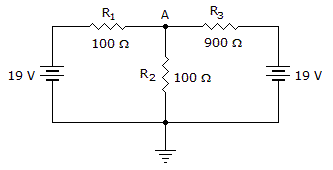# Electronics - Series-Parallel Circuits - Discussion

### Discussion :: Series-Parallel Circuits - Filling the Blanks (Q.No.3)

3.In the given circuit, the current flowing through R3 equals ______.

 [A]. 10 mA [B]. 21.11 mA [C]. 90 mA [D]. 100 mA

Explanation:

No answer description available for this question.

 Deepak Jr said: (Oct 4, 2016) How this? Explain me.

 Sapana M A said: (Sep 16, 2017) By applying KVL for both loops we get following equations. 200I1-100I2=19, -100I1+ 1000I2= -19, so we get I1=90mA. and I2=-10mA, So across R3 current I2 is flowing which is 10mA.

 Sapana Adhalli said: (Sep 20, 2017) 200 I1 - 100 I2= 19 --> equation 1. -100 I1 + 1000 I2 = -19 --> equation 2. solving equation 1 and 2 we get, I1 =90mA and I2 which is accross R3 that is I2 = -10mA.